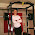### C code optimization benchmark

Steve Oualline talks about C code optimization on his book: Practical C Programming. I was curious about the real performance gains. The benchmark test results are at the end of the post.

How can this C code be optimized?
matrix1.c

#define X_SIZE 60
#define Y_SIZE 30
int matrix[X_SIZE][Y_SIZE];
void initmatrix(void)
{
int x,y;
for (x = 0; x < X_SIZE; ++x){
for (y = 0; y < Y_SIZE; ++y){
matrix[x][y] = -1;
}
}
}
void main()
{
initmatrix();
}
The first suggested optimization is to use the "register" qualifier for the indexes variables x and y:
matrix2.c

#define X_SIZE 60
#define Y_SIZE 30
int matrix[X_SIZE][Y_SIZE];
void initmatrix(void)
{
register int x,y;
for (x = 0; x < X_SIZE; ++x){
for (y = 0; y < Y_SIZE; ++y){
matrix[x][y] = -1;
}
}
}
void main()
{
initmatrix();
}
Then the optimization suggestion is to order the for loops so that the innermost for is the most complex:
matrix3.c

#define X_SIZE 60
#define Y_SIZE 30
int matrix[X_SIZE][Y_SIZE];
void initmatrix(void)
{
register int x,y;
for (y = 0; y < Y_SIZE; ++y){
for (x = 0; x < X_SIZE; ++x){
matrix[x][y] = -1;
}
}
}
void main()
{
initmatrix();
}
The most tricky to understand is changing Y_SIZE from 30 to 32. This will activate one feature of most C compilers that converts multiples by a power of  2 (2, 4, 8, ...) into shifts. This will result in performance gains when the computer is doing pointer arithmetic to access the correspondent memory address of matrix[x][y]. The compiler will change one multiplication operation into one shift operation which is cheaper.
matrix4.c
#define X_SIZE 60
#define Y_SIZE 32
int matrix[X_SIZE][Y_SIZE];
void initmatrix(void)
{
register int x,y;
for (y = 0; y < Y_SIZE; ++y){
for (x = 0; x < X_SIZE; ++x){
matrix[x][y] = -1;
}
}
}
void main()
{
initmatrix();
}
Reducing the number of loops and taking control of the pointer arithmetic is great performance optimization.
matrix5.c

#define X_SIZE 60
#define Y_SIZE 30
int matrix[X_SIZE][Y_SIZE];
void initmatrix(void)
{
register int index;
register int *matrix_ptr;
matrix_ptr = &matrix;
for (index = 0; index < X_SIZE * Y_SIZE; ++index){
*matrix_ptr = -1;
matrix_ptr++;
}

}
void main()
{
initmatrix();
}
Reducing the number of variables that are necessary to do the pointer arithmetic also improves performance:
matrix6.c
#define X_SIZE 60
#define Y_SIZE 32
int matrix[X_SIZE][Y_SIZE];
void initmatrix(void)
{
register int *matrix_ptr;
for (matrix_ptr = &matrix;
matrix_ptr <= &matrix[X_SIZE - 1][Y_SIZE - 1];
++matrix_ptr){
*matrix_ptr = -1;
}

}
void main()
{
initmatrix();
}
Looks like that there is nothing more to optimize. You can always write some assembly but it may not be good idea. The library function memset() can be used to fill a matrix. "Frequent used library subroutines like memset are often coded into assembly language and may make use of special processor-dependent tricks to do the job faster than could be done in C".
matrix7.c
#include
#define X_SIZE 60
#define Y_SIZE 30
int matrix[X_SIZE][Y_SIZE];
void initmatrix(){
memset (matrix, -1, sizeof(matrix));
}

void main()
{
initmatrix();
}
There is overhead in function call. It is possible to do better with macros.
matrix8.c
#include
#define X_SIZE 60
#define Y_SIZE 30
int matrix[X_SIZE][Y_SIZE];
#define initmatrix() \
memset (matrix, -1, sizeof(matrix));

void main()
{
initmatrix();
}
The improvements looks good, but how much efficient each optimizations are? I've measured it in clock cycles. And found that the optimization level is processor dependent.

For clock cycles, lower is better.

Results for: Intel(R) Core(TM) i7-2620M CPU @ 2.70GHz
 clock cycles times faster matrix1() 11102.151556 1 matrix2() 6400.36597 1.7346119906 matrix3() 6379.460394 1.740296337 matrix4() 5952.497506 1.8651249404 matrix5() 2154.262528 5.153574094 matrix6() 1907.350431 5.8207193474 matrix7() 792.123493 14.0156827239 matrix8() 780.254779 14.2288799182

Results for: Intel(R) Core(TM)2 Quad CPU    Q8400  @ 2.66GHz
 clock cycles times faster matrix1() 17175.114362 1 matrix2() 8153.467501 2.1064797719 matrix3() 8063.182452 2.1300664427 matrix4() 8497.82453 2.0211189701 matrix5() 4300.083046 3.9941355035 matrix6() 4321.695819 3.9741608575 matrix7() 1569.097383 10.945856228 matrix8() 1560.792718 11.0040969335

Results for: AMD Athlon(tm) 7750 Dual-Core Processor @ 2.7GHz
 clock cycles times faster matrix1() 25319.969906 1 matrix2() 10329.498185 2.4512294259 matrix3() 8558.934585 2.9583086136 matrix4() 9480.851235 2.6706430972 matrix5() 5544.608885 4.5665926003 matrix6() 5577.454075 4.5397002943 matrix7() 643.046753 39.3750062307 matrix8() 631.545791 40.0920570873

So, it is real! For Intel you can get 2 times faster performance by doing simple changes and not using pointer arithmetic. If you do take control of pointer arithmetic and trash some variables, the performance gain can go up to almost 6 times faster. The performance gain can reach 14 times faster by using ultra specialized subroutines. It is much better then I was expecting.

For AMD the use of the specialized functions can result in speedup of more than 40 times.

The clock cycle count is not an integer because the values shown are average mean of 256 measurements.

For the graphs that shows results in clock cycles, lower is better.C code optimization benchmark: Core i7C code optimization benchmark: Core 2 QuadC code optimization benchmark: Athlon X2

rdtscbench was used to do the benchmark testing. The source code is available here. The command line for rdtscbench was: "# ./rdtscbench 256 8". It is also available at: https://github.com/petersenna/rdtscbenchAnonymous said…
Peter,

Nice test, I've always liked this kinda tweak.

Just a comment. How about using a pre-computed value, like int bound = X_SIZE * Y_SIZE, instead of multiplication for every loop in 5 and 6?

TTV.Anonymous said…
Peter,

Nice test, I've always liked this kinda tweak.

Just a comment. How about using a pre-computed value, like int bound = X_SIZE * Y_SIZE, instead of multiplication for every loop in 5 and 6?

TTV.Peter said…
Hey TTV,

Can you do the test? It should not be difficult...

The tool I used for the benchmark is rdtscbench:
https://github.com/petersenna/rdtscbench

The matrix examples are on the file:
https://github.com/petersenna/rdtscbench/blob/master/matrix/matrix.c

You should be able to compile rdtscbench in any Linux machine.